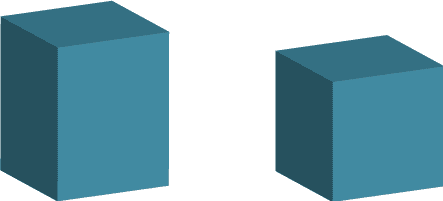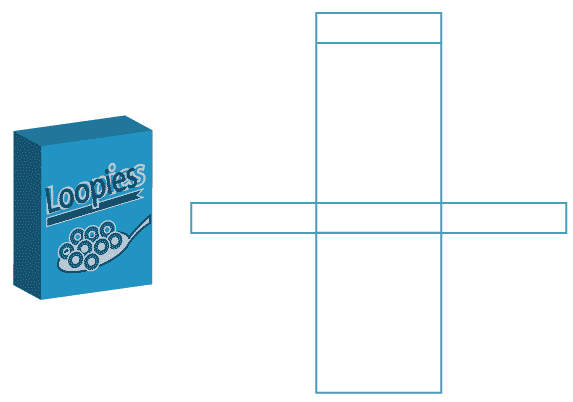### Rectangular prisms

A rectangular prism is a three-dimensional object with rectangles as all of its faces. If all the faces of the prism are squares, then the rectangular prism can also be called a cube.The net of a polyhedron is like an unfolded cardboard construction of that polyhedron. One example is the rectangular-prism cereal packet and its net shown here.A rectangular prism has 6 faces, 8 vertices (or corners) and 12 edges. To build a rectangular prism with construction materials, we would need 6 rectangles that join at the edges to make a closed three-dimensional shape or 12 edge pieces and 8 corner pieces to make a frame of a rectangular prism.
A cube or rectangular prism can also be called a hexahedron because it has six faces. Hexa means six.